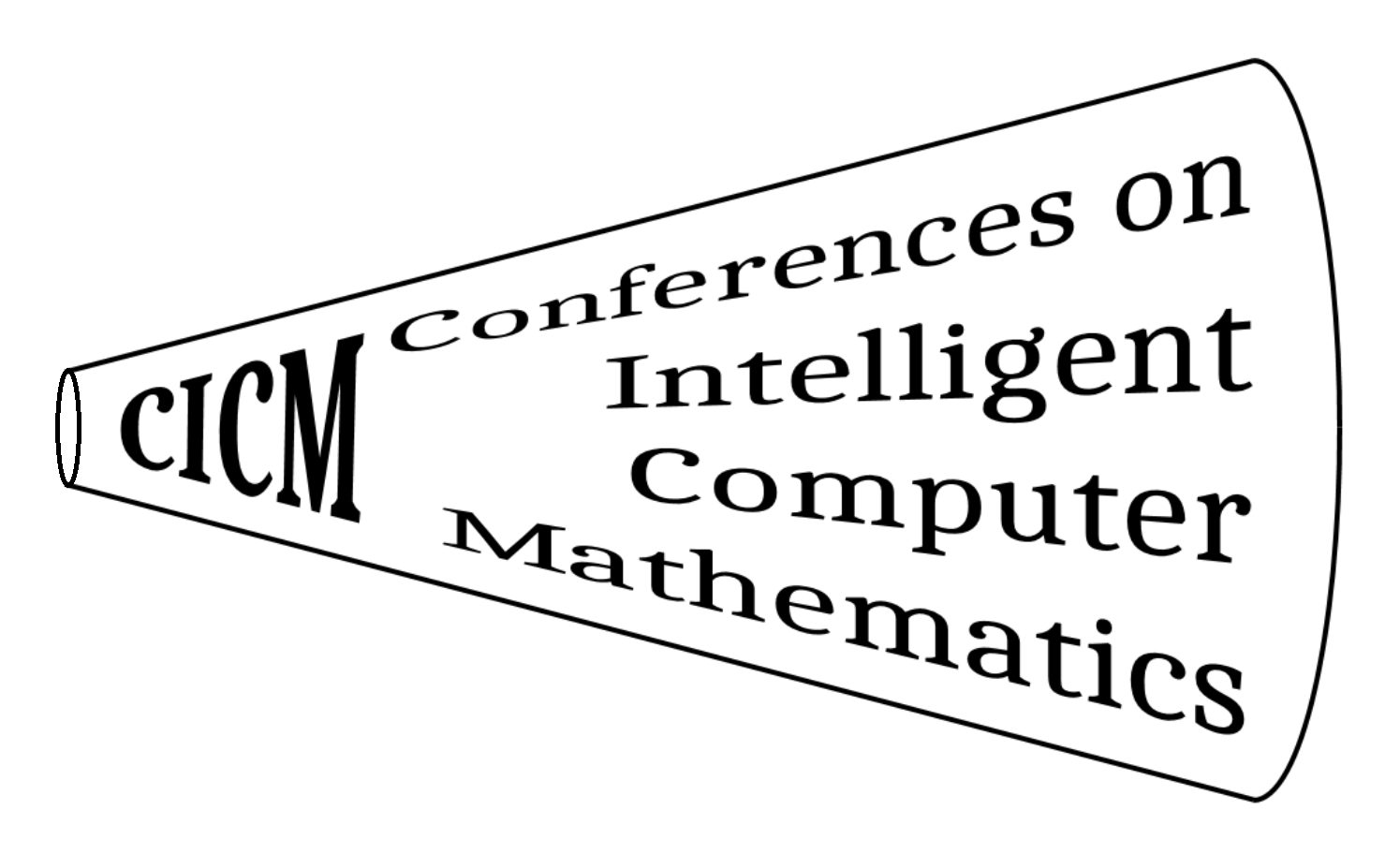You must enable JavaScript to use this site
• # Track: MKM (Chair: Bruce Miller)

Mathematical Knowledge Management is an interdisciplinary field of research in the intersection of mathematics, computer science, library science, and scientific publishing. The objective of MKM is to develop new and better ways of managing sophisticated mathematical knowledge, based on innovative technology of computer science, the Internet, and intelligent knowledge processing. MKM is expected to serve mathematicians, scientists, and engineers who produce and use mathematical knowledge; educators and students who teach and learn mathematics; publishers who offer mathematical textbooks and disseminate new mathematical results; and librarians and mathematicians who catalog and organize mathematical knowledge.

The track is concerned with all aspects of mathematical knowledge management. A non-exclusive list of important topics includes:

• Representations of mathematical knowledge
• ranging from formal to informal
• for mathematical formula or meta-knowledge about mathematics
• standards including MathML, OpenMath, Content Dictionaries
• presentation of mathematics on the web and elsewhere
• Creation and discovery of mathematical knowledge
• Authoring languages and tools, OCR
• Collaboration tools for mathematics
• Data mining, theory exploration
• Methods for managing, storing, discovering and manipulating mathematical knowledge
• Analysis and deduction systems (CAS, ATP)
• Mathematical search and retrieval; repositories and access
• Math assistants, tutoring and assessment systems
• Challenges and solutions for mathematical work-flows

The interests of the MKM Track of CICM necessarily, and intentionally, overlap those of other CICM tracks but generally with a broader point of view. Authors of papers dealing more specifically with Theorem Proving or Mathematical Libraries may consider submitting to the Calculemus or DML tracks, respectively. Work involving prototypes of or enhancements to systems may wish to submit to the Systems and Data track, possibly as a complementary paper.

• ### News